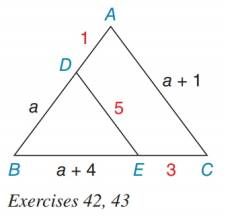Chapter 5.1, Problem 43EElementary Geometry For College St...

7th Edition
Alexander + 2 others
ISBN: 9781337614085

Solutions

Chapter
SectionElementary Geometry For College St...

7th Edition
Alexander + 2 others
ISBN: 9781337614085
Textbook Problem

In the figure, assume that a > 0. Prove that B D ⋅ D E ≠ B E ⋅ A C .To determine

To prove:

BDDEBEAC.

Explanation

It is given, BD = a, DE = 5, BE = a + 4 and AC = a + 1.

Substitute BD = a and DE = 5 in BDDE.

BDDE=5a     .....(1)

Substitute BE = a + 4 and AC = a + 1 in BEAC.

BEAC=(a+4)(a+1)

Still sussing out bartleby?

Check out a sample textbook solution.

See a sample solution

The Solution to Your Study Problems

Bartleby provides explanations to thousands of textbook problems written by our experts, many with advanced degrees!

Get Started

(t+6)(60)(60t+180)(t+6)2

Applied Calculus for the Managerial, Life, and Social Sciences: A Brief Approach

Convert the following percents to decimals. 35

Contemporary Mathematics for Business & Consumers

True or False: ln(a + b) = ln a + ln b.

Study Guide for Stewart's Single Variable Calculus: Early Transcendentals, 8th